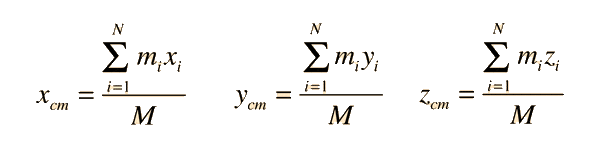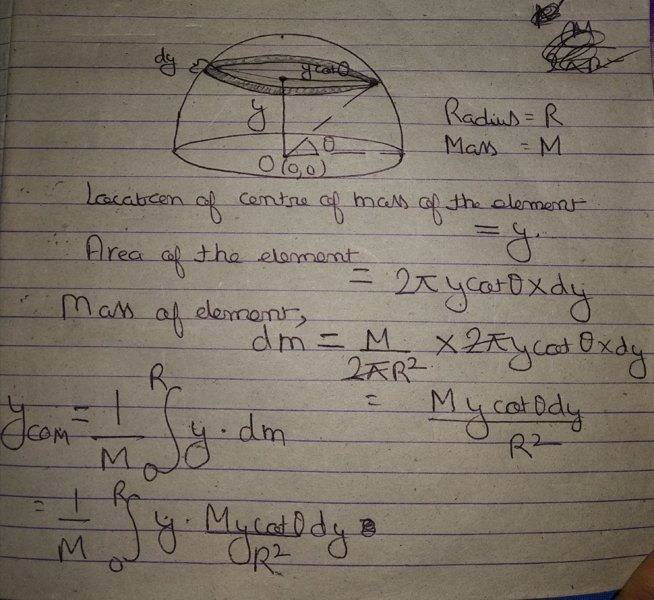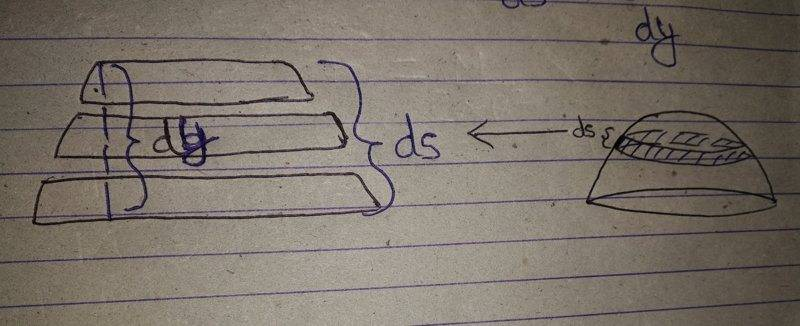# Need help in finding COM of a hollow hemisphere

• navneet9431
In summary, the student tries to calculate the COM of a hemisphere, but is unable to find the correct answer. He is then helped by a tutor who explains that integration is used to find the correct answer.f

Gold Member

## Homework Statement

Hey everyone,
I'm studying for my physics and came across a question for the COM of a hemisphere.I made my attempt to calculate the COM.

## Homework Equations## The Attempt at a Solution

I tried to calculate the y coordinate of COM this way,please go through it-I will be thankful for any help.

#### Attachments

##dy## in your drawing is not actually ##dy##. ##dy## would be a vertical line. That angled line ##ds## is longer, do you see that? Use ##\theta## to find its length. Then I think you'll get it.

##dy## in your drawing is not actually ##dy##. ##dy## would be a vertical line. That angled line ##ds## is longer, do you see that? Use ##\theta## to find its length. Then I think you'll get it.
Thanks but I can see how dy is a vertical line!
Can you send a rough diagram showing that?

Thanks but I can see how dy is a vertical line!
Can you send a rough diagram showing that?

##dy## is the thickness of the disc. ##ds## is the length of the edge. Do you understand?

•navneet9431
##dy## is the thickness of the disc. ##ds## is the length of the edge. Do you understand?
Ok!
So what should I use for calculating the area of the element?
Should I use dy?

Ok!
So what should I use for calculating the area of the element?
Should I use dy?

This should be in your book at some point. It'll explain why it works that way. But you need to use ##ds## or it won't give you the right answer.

•navneet9431
This should be in your book at some point. It'll explain why it works that way. But you need to use ##ds## or it won't give you the right answer.
This is the main problem!
My textbook nowhere explains why it works that way.
Can I get a bit of expalantion about why it works that way?

This is the main problem!
My textbook nowhere explains why it works that way.
Can I get a bit of expalantion about why it works that way?

Do you need a better book? It's not my policy to explain what a book could explain better anyway. Sorry.

Last edited:See the image.
These are the horizontal slices of the element ds as shown in the pic.
I think I have made the diagram correct as dy is the horizontal thickness and ds is the thickness of the edge.
So,if we see logically then it would be incorrect to calculate the area of the element this way, area of the element= 2*pi*y*cot(theta)*d(s) as the thickness of edge of each slice of the element marked on the hemisphere is different.
So calculating the area of the element this way must be wrong,Am I correct?

#### Attachments

2*pi*y*cot(theta)*d(s)
I think you mean 2πx (the radius is horizontal in the diagram) and it should not be cot. ds is the hypotenuse.